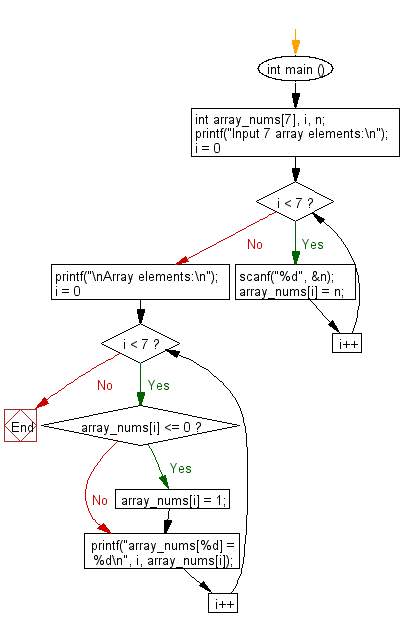﻿ C : Replace every negative or null element of an array by 1

# C Exercises: Replace every negative or null element of an array by 1 and print the array elements

## C Basic Declarations and Expressions: Exercise-124 with Solution

Write a C program that reads an array of integers (length 7), replaces every negative or null element with 1 and prints the array elements.

Sample Solution:

C Code:

``````#include <stdio.h>

int main () {
int array_nums, i, n;

// Prompt user for input
printf("Input 7 array elements:\n");

// Loop to read 7 integer values from the user and store them in the array
for (i = 0; i < 7; i++) {
scanf("%d", &n);
array_nums[i] = n;
}

// Print a message indicating the array elements will be displayed
printf("\nArray elements:\n");

// Loop to print each element of the array
for (i = 0; i < 7; i++) {

// Check if the element is less than or equal to 0
if (array_nums[i] <= 0) {
array_nums[i] = 1; // Set the element to 1 if it's less than or equal to 0
}

// Print the array element and its value
printf("array_nums[%d] = %d\n", i, array_nums[i]);
}

return 0; // End of program
}
``````

Sample Output:

```Input 7 array elements:
15
12
-7
25
0
27
53

Array elements:
array_nums = 15
array_nums = 12
array_nums = 1
array_nums = 25
array_nums = 1
array_nums = 27
array_nums = 53
```

Flowchart:C programming Code Editor:

What is the difficulty level of this exercise?

Test your Programming skills with w3resource's quiz.

﻿